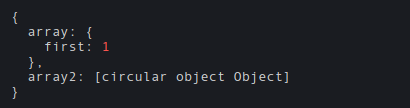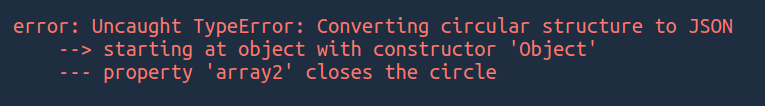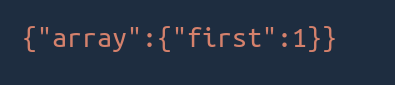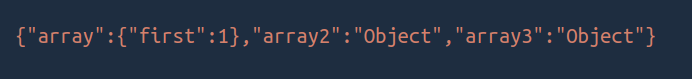# How to print a circular structure in a JSON like format using JavaScript ?

• Last Updated : 09 Feb, 2021

The circular structure is when you try to reference an object which directly or indirectly references itself.

Example:

Hey geek! The constant emerging technologies in the world of web development always keeps the excitement for this subject through the roof. But before you tackle the big projects, we suggest you start by learning the basics. Kickstart your web development journey by learning JS concepts with our JavaScript Course. Now at it's lowest price ever!

`A -> B -> A   OR   A -> A`

Circular structures are pretty common while developing an application. For Example, suppose you are developing a social media application where each user may have one or more images. Each image may be referencing its owner. Something like this:

```{
User1: {
Image1:{
URL: 'Image Url',
Owner: User1 (object)
},
Image2:{
URL: 'Image Url',
Owner: User1 (object)
}
}
}```

Here you can easily resolve this by passing the user id to Owner instead of the user object.

Passing such objects to JSON.stringify() results in ‘Converting circular structure to JSON Error’.

Let’s take an example and try to resolve this issue.

Example:

## Javascript

 `var` `object = {};``object.array = {``'first'``:1};``object.array2 = object;`` ` `console.log(object);`

The output should look something like this:If we pass the above object to JSON.stringify() then this will result in the following error:To resolve this we can pass another parameter to JSON.stringify() which is actually a function. And we can handle the object however we want inside the function. It takes two parameters, the key and the value that is being stringified. It gets called for each property on the object or array being stringified. It should return the value that should be added to the JSON string.

Let’s create a function named circularReplacer.

```const circularReplacer = () => {

// Creating new WeakSet to keep
// track of previously seen objects
const seen = new WeakSet();

return (key, value) => {
// If type of value is an
// object or value is null
if (typeof(value) === "object"
&& value !== null) {

// If it has been seen before
if (seen.has(value)) {
return;
}

// Add current value to the set
}

// return the value
return value;
};
};```

Explanation:

• The above function will first create a WeakSet to keep track of previously seen objects. WeakSet in JavaScript is used to store a collection of objects. It adapts the same properties of that of a set i.e. does not store duplicates. Read more above WeakSet here.
• Checks if the type of value is an object and the value is not null. Then checks if it has been seen before. If yes then just return. if not then add it to the set.
• Instead of just return nothing when an object has been seen. We can return even more useful information, for example, return ‘Object’, which will tell us that value of this will create a circular structure.
• If the type of value is not an object or the value is null. Then simply return the value.

Example 1:

## Javascript

 `var` `object = {};``object.array = {``'first'``:1};``object.array2 = object;`` ` `const circularReplacer = () => {`` ` `    ``// Creating new WeakSet to keep ``    ``// track of previously seen objects``    ``const seen = ``new` `WeakSet();``     ` `    ``return` `(key, value) => {`` ` `        ``// If type of value is an ``        ``// object or value is null``        ``if` `(``typeof``(value) === ``"object"` `                   ``&& value !== ``null``) {``         ` `        ``// If it has been seen before``        ``if` `(seen.has(value)) {``                 ``return``;``             ``}``              ` `             ``// Add current value to the set``             ``seen.add(value);``       ``}``        ` `       ``// return the value``       ``return` `value;``   ``};``};`` ` `var` `jsonString = JSON.stringify(``         ``object, circularReplacer());``console.log(jsonString);`

Output:Note: If we just return when seeing a circular structure that key will not get added to the output string.

Example 2: Let’s return a string instead of nothing.

## Javascript

 `var` `object = {};``object.array = {``'first'``:1};``object.array2 = object;``object.array3 = object.array2;`` ` `const circularReplacer = () => {`` ` `    ``// Creating new WeakSet to keep ``    ``// track of previously seen objects``    ``const seen = ``new` `WeakSet();``     ` `    ``return` `(key, value) => {`` ` `        ``// If type of value is an ``        ``// object or value is null``        ``if` `(``typeof``(value) === ``"object"` `                  ``&& value !== ``null``) {``         ` `        ``// If it has been seen before``        ``if` `(seen.has(value)) {``                 ``return` `'Object'``;``             ``}``              ` `             ``// Add current value to the set``             ``seen.add(value);``       ``}``        ` `       ``// return the value``       ``return` `value;``   ``};``};`` ` `var` `jsonString = JSON.stringify(``       ``object, circularReplacer());``console.log(jsonString);`

Output:My Personal Notes arrow_drop_up